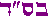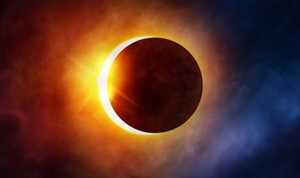D.A.F. >   Useful Resources > Emunah Issues > Torah Numerology[print version ]         [feedback ]
<<Previous: Wisdom = Yud

Divine Symmetries

divine symmetries in Genesis 1:1, Prime numbers, and PiThe Torah opens with the second letter Beit - a letter symbolizing plurality and duality. It consists of two parallel lines and one connecting line. Beit is a summary of the whole Torah. The top line represents Heaven (the spiritual) while the bottom line represents Earth (the physical). The connecting line is man's job to connect the two. This is the purpose of creation (Vilna Gaon).

The world was created with the Beit (Midrash Otyot d'Rabbi Akiva-Beit). Thus, we find duality permeates the universe at every level. In physics, we find every charged particle has a corresponding anti-particle with all properties reversed. All particles (including light) also have a mysterious dual wave/particle nature at the fundamental quantum level.In our human domain we find most creatures, including man, have a mirror (bilateral) symmetry. Namely, the creature can be cut into two mirror image halves identical to our eye. Other examples of duality: male/female, sun/moon, good/evil, body/soul, Heaven/Earth, and many more. All this is the signature of the Beit.

The Beit also represents the second of the Ten Sefirot used to create the world - the Sefira of Wisdom (Rabeinu Bechaye, Ber.1:1). The 10 Sefirot (spiritual divine lights) were divided into two groups of 3/7. Let us examine if this signature of duality is reflected mathematically.

As before the opening verse in the Torah has Gematria of 2701.

 Gematria of First Verse בְּרֵאשִׁ֖ית בָּרָ֣א אֱ-לֹהִ֑ים אֵ֥ת הַשָּׁמַ֖יִם וְאֵ֥ת הָאָֽרֶץ 913 203 86 401 395 407 296 Total=2701

The first example of duality is as we saw already:
• 2701 = 37 × 73 (mirror reflection)

• secondly, if we add 2701 to its own mirror reflection:

2701 + 1072 = 3773

The original 37/73 prime factors are revealed in this sum. (I tested the first 100 million+ numbers by computer and none other than 2701 have these two symmetries)

• Another symmetry: if we multiply the 7 word Gematrias:
296×407×395×401×86×203×913
=304,153,525,784,175,760 (huge number indeed)

304+153+525+784+175+760= 2701 (same as Gematria!)
(3+0+4)
(=7)
+(1+5+3)
(=9)
+(5+2+5)
(=12)
+(7+8+4)
(=19)
+(1+7+5)
(=13)
+(7+6+0)
(=13)
= 73
(7)+(9)+(1+2)+(1+9)+(1+3)+(1+3)= 37
(again 73 and 37 the prime factors of 2701!)

G-d's Name

Interestingly, the Gematria of G-d's Name (the Tetragramaton) is 26:
26 × 62 (mirror reflection) = 1612
and 1612 + 2161 = 3773 (remember these numbers)

Prime Numbers

Prime numbers are prime basic structures of creation.

They are defined as numbers which cannot be divided by anything except 1 and themselves. Mathematicians categorize "2" as the first prime number (1 is in a category of its own. "2" is also the only even prime number, since all other even numbers are divisible by 2 and thus not prime).

Let us examine their mysterious connection to the Torah's opening verse.

(as before 2701 = 37×73)
Here are the first prime numbers until 73 (see table on the left).
 Prime Numbers Order# Prime# 1st 2 2nd 3 3rd 5 4th 7 5th 11 6th 13 7th 17 8th 19 9th 23 10th 29 11th 31 12th 37 13th 41 14th 43 15th 47 16th 53 17th 59 18th 61 19th 67 20th 71 21st 73

37 is the 12th prime number

73 is the 21st prime number

Note again the mirror symmetry in 12/21.

Note also that 12/21 are the very first pair of numbers with mirror symmetry.

Interestingly, the 12/21 prime indexes also have a mirror symmetry in their squares:

122 = 144 and 212=441

note the perfect mirror symmetry in 144/441

441 is also the Gematria of אמת = Truth

Our sages said the world was created with Truth, i.e. pure, absolute wisdom (Baal HaTurim Gen.1:1). In the lowest physical level, this manifests as the wisdom of mathematics.

73 represents wisdom as we saw. Thus, 21 is also symmetrical with 73 in its Gematria meaning. For "Truth" and "Wisdom" are synonymous here.

אמת (truth) = 441 = 3×7×7×3 (note again the 37/73)

Prime Numbers of Opening Verse

Observe the following incredible perfection.

 Gematria of First Verse בְּרֵאשִׁ֖ית בָּרָ֣א אֱ-לֹהִ֑ים אֵ֥ת הַשָּׁמַ֖יִם וְאֵ֥ת הָאָֽרֶץ 913 203 86 401 395 407 296 Total = 2701 = 37×73

 Prime Numbers of First Verse The 913th Prime = 7127 The 203rd Prime = 1237 The 86th Prime = 443 The 401st Prime = 2749 The 395th Prime = 2711 The 407th Prime = 2797 The 296th Prime = 1949 Total Sum = 2161st Prime (19013) 2161 + 1612 = 3773

Note that there is absolutely no logical reason of any kind for prime numbers to be related to their indexes.

There is no mathematical formula for obtaining prime numbers. They are found by trial and error.

Hebrew Alphabet

The Hebrew letters are the "prime number" building blocks of creation.

In the Talmud: "Betzalel knew how to join the letters with which Heaven and Earth were created" (Berachot 55a).
Rashi comments: G-d created the world by joining the Hebrew letters in various combinations.

The Hebrew alphabet is composed of 22 letters and 5 end letters. The most symmetric way to arrange them is in groups of three letters. This arrangement is known as Ayak Bachar.

note that in this arrangement each group is a multiple of 37×3 (37=wisdom!). 37 is the only number with this sequential repetitive digit property.

 Hebrew Alphabet Groups א י ק = 111 = 37×3×1 ב כ ר = 222 = 37×3×2 ג ל ש = 333 = 37×3×3 ד מ ת = 444 = 37×3×4 ה נ ך = 555 = 37×3×5 ו ס ם = 666 = 37×3×6 ז ע ן = 777 = 37×3×7 ח פ ף = 888 = 37×3×8 ט צ ץ = 999 = 37×3×9

Their prime number indexes are:

 Prime Numbers of Groups The 111th Prime = 607 The 222nd Prime = 1399 The 333rd Prime = 2239 The 444th Prime = 3119 The 555th Prime = 4019 The 666th Prime = 4973 The 777th Prime = 5903 The 888th Prime = 6907 The 999th Prime = 7907 Total Sum   = 37073 (incredible!)

Pi Positions

The circle is the most prime geometric figure and is defined by Pi.

Indeed, the creation began as a "dot" and expanded out as a perfect circle (Etz Chaim 1).

Here are the first positions in Pi of the Hebrew alphabet groups.
(these and the primes discovered by Leo Tavares)

 Locations in Pi "111" first appears in Pi : 153rd digit "222" first appears in Pi : 1735th digit "333" first appears in Pi : 1698th digit "444" first appears in Pi : 2707th digit "555" first appears in Pi : 177th digit "666" first appears in Pi : 2440th digit "777" first appears in Pi : 1589th digit "888" first appears in Pi : 4751th digit "999" first appears in Pi : 762nd digit Total Pi Locations   = 16012 (+21061 = 37073)!!

For example:
 First Appearances in Pi Pi=3. 14159265358979323846264338327950288419716939937510 58209749445923078164062862089986280348253421170679 82148086513282306647093844609550582231725359408128 48111745028410270193852110555964462294895493038196 44288109756659334461284756482337867831652712019091 45648566923460348610454326648213393607260249141273 72458700660631558817488152092096282925409171536436 78925903600113305305488204665213841469519415116094 33057270365759591953092186117381932611793105118548 07446237996274956735188575272489122793818301194912 98336733624406566430860213949463952247371907021798 60943702770539217176293176752384674818467669405132 00056812714... "111" appears starting at the 153rd digit "222" appears starting at the 1735th digit etc. (see above)

Another (of many hints of Pi in the Hebrew Letters and first verse):
If we sum the inverses of all the Hebrew letters:
1+1/2+1/3+1/4+1/5+1/6+1/7+1/8+1/9+1/10+1/20+1/30+1/40+1/50+1/60+1/70+1/80+1/90+1/100+1/200+1/300+1/400+1/500+1/600+1/700+1/800+1/900
=3.14015 (within 0.046 percent of Pi - M.Glazerson)

 To summarize: 2701 = 37×73 note mirror symmetry 2701+1072 = 3773 note prime factors revealed Words multiplied = 2701 sum of thousands Words multiplied = 73,37 first and second digit sums Third digit sum = 26 26×62=16121612+2161=3773 37th Prime = 12 73rd Prime = 21 12/21 mirror symmetry 122 = 144 212 = 441 144/441 mirror symmetry 73 = wisdom 212 = Truth Truth/Wisdom similar 212 = 3×7×7×3 1st verse Primes = 2161st Prime 2161+1612=3773 Hebrew Primes = 37073 Hebrew Pi Locations = 16012 21061+16012=37073

Other: as we will see, the first verse is symmetric around its last 2 words which have Gematria of 703 (same as the sum of thousands for 2701 and many other interesting things).
• as we will see 703=19×37 has the same double mirror symmetry as 2701=37×73 in the base 7 number system.

• The 37073rd prime = 441703 - (441="Truth" as above, 703=last 2 words).

• The number of integers between the 703rd prime (5303) and the 2701st prime (24317) = 2161st prime (19013) - same as the primes of the first verse!

• As before, the Gematria of G-d's Name is 26. 26×62=1612 and 1612+2161=3773

Center Points

Center points are an important property in the Genesis 1:1 mathematical structure.

What is the center point of 2701?

Center Point
1351 is the exact center point of 2701

 1--------------------2701 ^1351

i.e. for 2701 dots, 1351 is the middle dot. Let us dig deeper..

Center Point
676 is the exact center point of 1351

 1--------------------1351 ^676

Thus, the center point of the center point of 2701
= 676 = 262 (26 is G-d's Name)
This is also the final center point of 2701 since only odd numbers have center points.

How about 2161? What is its center point? (we have seen this number and its mirror reflection 1612 earlier)

Center Point
1081 is the exact center point of 2161

 1--------------------2161 ^1081

i.e. for 2161 dots, 1081 is the middle dot. Let us dig deeper..

Center Point
541 is the exact center point of 1081

 1--------------------1081 ^541

Thus, the center point of the center point of 2161 is 541 = Yisrael (ישראל).

Also interesting:
 2701 is the center point of 5401 (5401 is similar to 541) 271 is the center point of 541 (271 is similar to 2701)

We will see this number 541 (Yisrael) pop up again in many interesting ways, be'H.

>> Next: Pi and GenesisFootnotes
•  second digit sum means reducing the digit sum a second time as follows:
304=3+4=7, 153=1+5+3=9, 525=12=1+2=3, 784=19=1+9=10, 175=13=1+3=4, 760=13=1+3=4
•  the mathematical facts on the prime and pi indexes were discovered by Leo Tavares. He told me it is fine to use his findings and asked to be cited as the discover. return to text
•  Notice also, the difference between 2161 and 2701 = 540
And likewise, the difference between their reflections, 1612 and 1072 = 540
540 forms a Star of David figurate with 12 Triangles of 45 (Adam) around a center point. see end of section "Geometric Properties" return to text

Mirrored from dafyomireview.com/543
contact